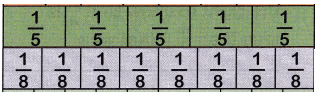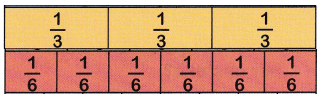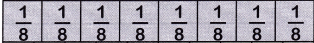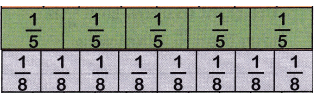# McGraw Hill Math Grade 4 Chapter 7 Lesson 5 Answer Key Using Benchmarks to Compare Fractions

Practice the questions of McGraw Hill Math Grade 4 Answer Key PDF Chapter 7 Lesson 5 Using Benchmarks to Compare Fractions to secure good marks & knowledge in the exams.

## McGraw-Hill Math Grade 4 Answer Key Chapter 7 Lesson 5 Using Benchmarks to Compare Fractions

Compare

Compare each pair of fractions. Write <, >, or =. Use $$\frac{1}{2}$$ or $$\frac{1}{4}$$ as benchmarks.

Question 1.
$$\frac{1}{3}$$ < $$\frac{3}{4}$$
$$\frac{1}{3}$$ < $$\frac{3}{4}$$
Explanation:
$$\frac{1}{3}$$ < $$\frac{1}{2}$$
$$\frac{3}{4}$$ > $$\frac{1}{2}$$
so,
$$\frac{1}{3}$$ < $$\frac{3}{4}$$

Question 2.
$$\frac{2}{5}$$ ____ $$\frac{3}{8}$$
$$\frac{2}{5}$$ > $$\frac{3}{8}$$
Explanation:$$\frac{2}{5}$$ > $$\frac{1}{4}$$
$$\frac{3}{8}$$ > $$\frac{1}{4}$$
According to the chart $$\frac{2}{5}$$ is greater than $$\frac{3}{8}$$

Question 3.
$$\frac{2}{4}$$ ____ $$\frac{2}{6}$$
$$\frac{2}{4}$$ > $$\frac{2}{6}$$
Explanation:According to the chart $$\frac{2}{4}$$ is greater than $$\frac{2}{6}$$

Question 4.
$$\frac{2}{3}$$ ____ $$\frac{3}{6}$$
$$\frac{2}{3}$$ > $$\frac{3}{6}$$
Explanation:$$\frac{2}{3}$$ is greater than $$\frac{3}{6}$$ according to the chart.

Question 5.
$$\frac{1}{3}$$ ____ $$\frac{2}{6}$$
$$\frac{1}{3}$$ = $$\frac{2}{6}$$
Explanation:$$\frac{1}{3}$$ is equal to $$\frac{2}{6}$$ according to the chart.Question 6.
$$\frac{4}{8}$$ ____ $$\frac{5}{8}$$
$$\frac{4}{8}$$ ____ $$\frac{5}{8}$$
Explanation:$$\frac{4}{8}$$ is lesser than $$\frac{5}{8}$$
according to the chart.

Question 7.
A school flag is $$\frac{3}{8}$$ gold and $$\frac{1}{5}$$ brown. Is more of the flag colored gold or brown?$$\frac{3}{8}$$ is greater than $$\frac{1}{5}$$